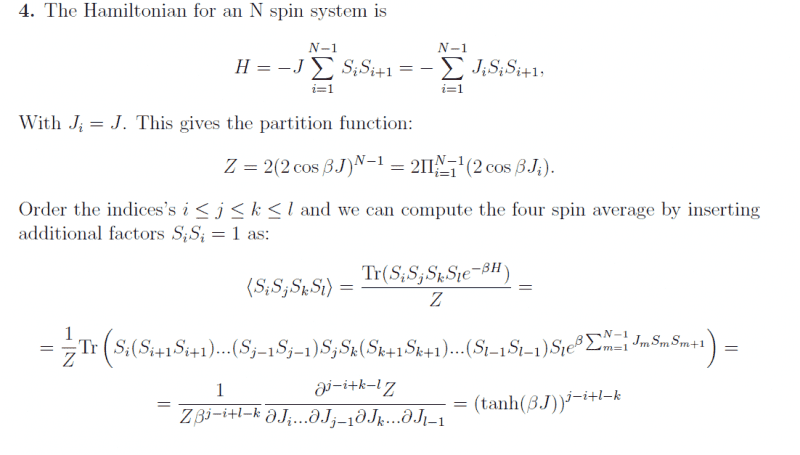# Statistical mechanics, spin average

This is an exercise from an old examination that im trying to understand by following the given solution to this problem. The exercise is about to calculate the four spin average <SiSjSkSl> and the indices i,j,k and l, given a Hamiltonian H.

Solution:The problem is that i can't follow the last two steps in this given solution. I understand how the partition function Z is obtained and how they express the four spin average as the trace of the four spins and the "density operator" (e^(-beta*H)/Z). But im very confused about how they continue to the next last and last rows.

I'm thankful for explanations!